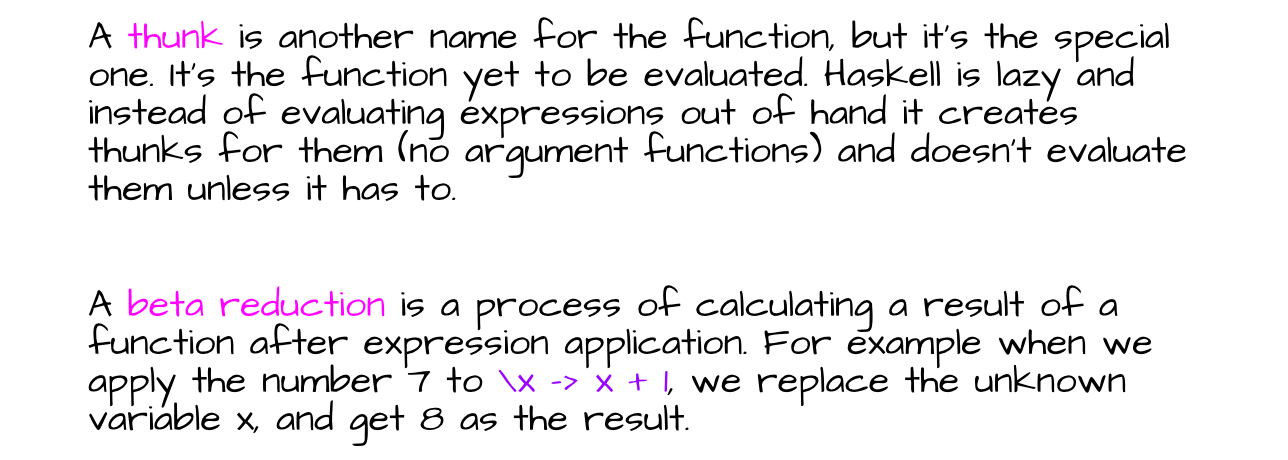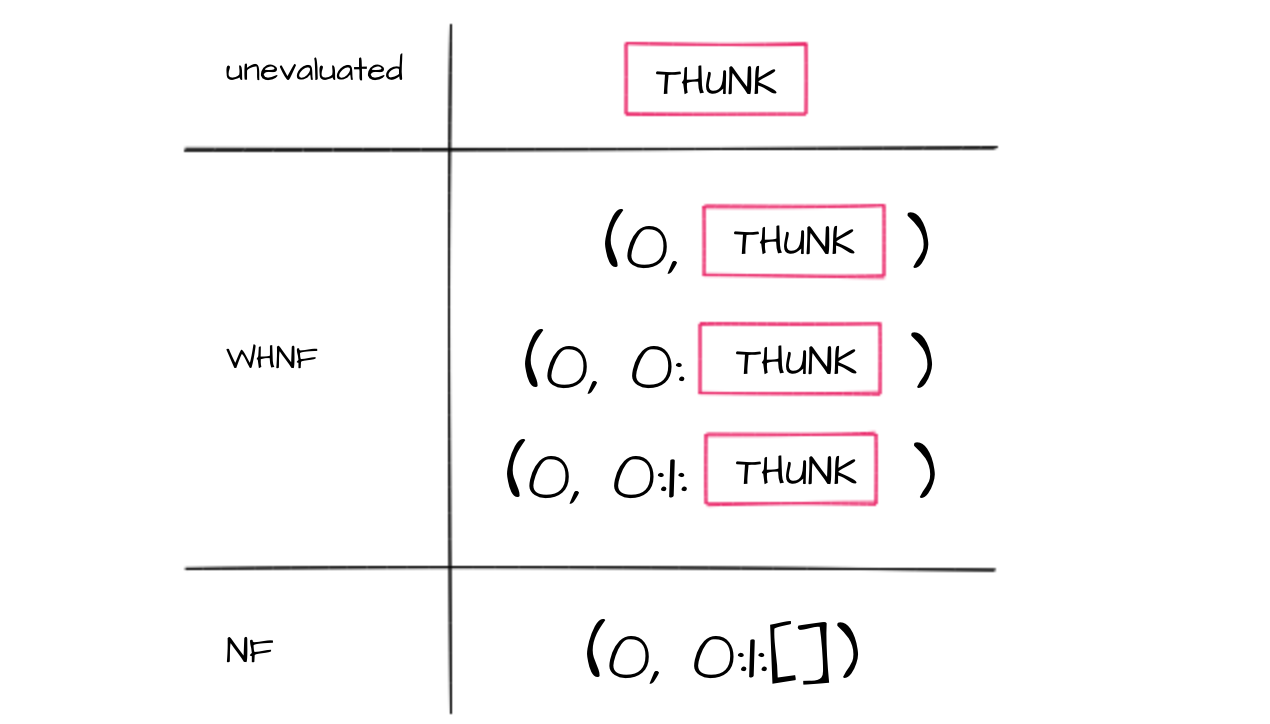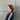# Brief normal forms explanation with Haskell

Before we start to befriending normal forms, there are some basic terms I’d like to briefly introduce.### Reduced Normal Form

We’re going to start with my favourite. Why is that my best one? Because of its tricky definition. Stay focus.

Expression A has r-nf if there exists term B obtained from A that is in r-nf and B is in rn-f where there’s no term A such A can be obtained from B and A has r-nf.

Take your time reading it couples of times. I took me a while to see the difference between having and being when I read about it in the internet.

So, what does it mean exactly? Consider some expression A, which has an r-nf. It means than A can be transformed using reduction rules to another expression B, such that it cannot be transformed further in any way — there’s no possible reduction for the term B. In other words B is reduction-free. Let’s look at the example below:

``f = \x -> (\y -> y) x``

The above term has r-nf, because it can be transform using beta-reduction to the following one:

``f = \x -> x``

which on the other hand cannot be reduced in any way, which means it’s in r-nf.

You can think of the hnf as of the simplest form of the particular expression. The head normal form (or just normal form) is the same as reduced normal form. In other words — it has no thunks inside.

An expression in normal form is fully evaluated, and no sub-expression could be evaluated any further.

For example these expressions are all in normal form:

``````7
1:2:[]
\x -> (x + 1)``````

In general Haskell makes no use of head normal form, because of its laziness. But HNF can be obtained with some poking around inside the function bodies. In haskell prelude there’s function seq that introduces some strictness to a Haskell program. seq:: a ->b -> b takes two arguments of any type, and returns the second. However, it also evaluates the outermost part of the first one. But all sub-expressions stay as they were, so there’s no certainty in obtaining a normal form. That’s where deepseq comes in handy. This package provides methods for fully evaluating data structures (deep evaluation) and is the way to bring full strictness to the program.

First, let’s look at some examples of the expressions that are in weak normal form (WHNF).

``````(1 + 1, 1 + 2)
'h' : ("e" ++ "llo")
\x -> 2 + 2``````

In the first two examples the outermost part (the head) is data constructor and in the last one the head is a lambda abstraction. And it’s the gist of WHNF.

An expression is in weak head normal form when the outermost part has been evaluated to the data constructor or lambda abstraction. Sub-expressions may not have been evaluated. If all the sub-expressions would be evaluated then the expression would be in normal form. The conclusion is: every normal form expression is also in weak normal form. The opposite is not always the true.

To determine whether an expression is in weak head normal form, we only have to look at the outermost part of the expression. For example these expressions are not in weak head normal form:

``````1 + 2
(\x -> x + 1) 2``````

The first expression is an application of (+) and the second is a function a function application.

In head normal form part of this article I wrote that normal form expressions have no thunks inside. In weak head normal form thunks are admissible unless they aren’t in the outermost part of the expression.### Beta Normal Form

An expression is in beta normal form when no beta reductions can be applied.

It means that every function application were properly evaluated and there’s nothing to reduce.

For example, suppose we apply the following function to some value:

``(\x -> x + y) 7``

To get the result, we have to substitute 7 for every occurrence of x, and so the application of the function is reduced to the result that is in beta normal form:

``7 + y``

Published under  on .### Aleksandra

Hi! I'm Aleksandra, a software developer based in Wrocław.